# Solving Equations Part B: False Position and Backtracking (30 minutes)

## Session 6, Part B

### In This Part

• False Position
• Backtracking

### False Position

The solutions to mathematical problems often involve writing and solving equations. We generally think of solving equations by representing quantities with variables. Early Egyptians did not have symbolic notation, however, so they invented a technique called false position to solve mathematical problems.
Note 4

The Egyptians used false position to solve number puzzles. A modified version of false position was used by Diophantus to solve problems with squared variables and was still taught in the Renaissance for proportion problems.

False position begins by selecting a convenient answer or making an educated guess, one that makes the calculations of the problem simpler. It does not have to be the correct answer. After calculating the result from the convenient answer, a false position problem is solved by using the result to determine how to adjust the convenient answer to make it correct.

Here’s an example from the Rhind papyrus, an ancient Egyptian scroll containing mathematical tables and calculations:To use the method of false position, start by selecting a convenient answer. Let’s pick 4. Why 4? It simplifies the calculation: 4, plus 1/4 of itself (which is 1), equals 5.

Next, use the result to determine how to adjust the convenient answer. We got 5 as our result, and we wanted 15. The number to multiply by 5 (the result we got) to get 15 (the result we want) is 3.

So, multiply 4 (the convenient answer we started with) by 3 to get the correct answer, which is 12.

There are a number of similar false position problems in the Rhind papyrus. Try to solve the following problem using the method of false position:

### Problem B1

A quantity and its 1/7 added together become 32. What is the quantity?

Tip: Start by assuming a “convenient” value for the solution, one that makes the “1/7” portion of the problem easier. Then, decide how to adjust the answer you got to make 32, the answer you want. “Its 1/7” means 1/7 of the original quantity.

### Problem B2

Consider the following equation: 4[3(2n – 4) / 6] = 8. Think of as many strategies as you can for solving this equation.
Note 5

Tip: False position will work here. You might also try writing the steps of the equation as an algorithm of function machines, and use a diagram to find the value of n. Have a look at Session 3, Part C.

### Backtracking

Problem B3

Using the equation from Problem B2:

1. Create a flow chart for the equation. Consider n as the input and 8 as the output.
2. Work backwards from your flow chart to find the value of n that produces 8 as an output.

This method of solving equations is called backtracking. Backtracking involves “undoing” operations to work backwards from the output to the input.

 Video Segment In this video segment, Professor Cossey draws a flow chart for the equation in Problem B2, then demonstrates the method of backtracking. You can choose to do Problem B3 before or after watching the video segment. You can first try to do the problem on your own, then use the video segment to reinforce what you’ve learned. Or you can watch the video segment before doing the problem to help you get started making flow charts or doing the method of backtracking. How are flow charts similar to the function machines you created in Session 3, Part C? You can find this segment on the session video, approximately 9 minutes and 9 seconds after the Annenberg Media logo.

Problem B4

Solve each of the following using backtracking:

1. 5(b / 2 – 3) = 20
2. 7(n + 1) / 2 = 14

Problem B5

Can you find an equation that cannot be solved by backtracking?

Tip: Think about whether a function machine’s operation could always be “undone.” You might also look for problems in Part C that would be difficult to solve by backtracking.

Problem B6

I’m thinking of a number. When I subtract 3 from my number, multiply the result by 8, then divide this result by 3, I get 16. What is my number?

Problem B7

Do problems that can be solved by backtracking have anything in common?

Problem B8

Look at the toothpick pattern below. One of the stages needs 112 toothpicks to form the pattern. Can you use backtracking to find out which stage it was?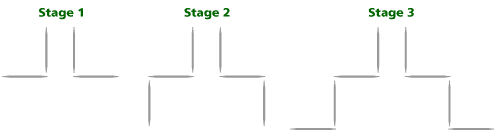Tip: A similar problem appears in Session 2, Part B. If you find a formula for the number of toothpicks at a given stage, you can backtrack using that formula.

#### Take It Further

Some equations lend themselves to a process called covering up. Covering up takes a complex equation and changes it into a series of one-step equations. For example, let’s say we wanted to solve the equation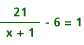A solution by covering up would begin by covering the most complicated expression in the equation (in this case, 21 / (x + 1) is the expression). Then the equation reads (covered) – 6 = 1, an equation that is solved quickly. Now we know that 21 / (x + 1) = 7. To continue, cover up the most complicated expression in the new equation, which is x + 1. The equation reads 21 / (covered) = 7. Now we know that x + 1 = 3, so x must be 2.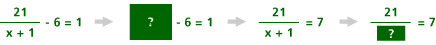#### Take It Further

Problem B9

1. Solve Problem B2 by the method of covering up: 4[3(2n – 4) / 6] = 8.
2. Solve the following equation by covering up: 3(12 / [x – 5]) + 1 = 13.

#### Take It Further

Problem B10

On Monday, the produce manager stocked his store’s display case with 80 heads of lettuce. By the end of the day some heads of lettuce had been sold. On Tuesday, the manager counted the number of heads of lettuce that were left and decided to add an equal number of heads of lettuce, thereby doubling the leftovers. By the end of the day he had sold the same number of heads of lettuce as on Monday.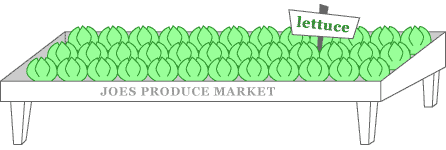On Wednesday, the manager decided to triple the number of heads of lettuce that had been left in the case. He sold the same number of heads of lettuce that day, too. At the end of the day, though, there were no heads of lettuce left.

How many were sold each day?

Describe the strategies you used to solve this problem.

Tip: Which of the methods in this part would be useful here? What would be a useful variable? Don’t forget: The same number of heads of lettuce are sold each day.

### Notes

Note 4

In this section, we’ll look at informal strategies for solving equations.

The method of false position is introduced as a specific case of guess, check, and improve. We will also attempt to solve equations by backtracking.

Groups: Start off by discussing the method of false position, using the historical background and the example given in the course.

The method of false postion is a case of guess, check, and improve. Consider why it works, and if it works in every situation. Groups can work in pairs on Problems B1 and B2.

Note 5

Now move on to other strategies for solving equations. Begin by asking one participant to think of a number.

Groups: A facilitator or another group member should lead this activity. The leader begins by asking one person to think of a number. That person should write the number on a piece of paper and hold it up for the rest of the group to see, without showing it to the leader. Then, the leader asks the group to volunteer different operations with numbers, such as multiply by 2, subtract 4, add 1, etc. The leader writes these on the board as a flowchart, including about five or six steps. The picture will look something like this: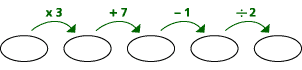The leader then asks the groups to run their number through the flowchart, and only reveal their answers. The group can discuss their ideas for figuring out the input. The leader then works backwards, generating a drawing on the board that looks something like this: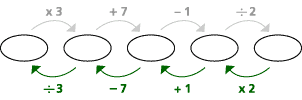Backtracking is a method that can be used before students know anything about formal equation solving. It simply requires that any operations be “undone” to work backwards from the output to the input.

Groups: Work in pairs on Problems B3-B10. In Problem B4, recognize that in order to solve a problem using backtracking, the variable must appear on one side of the equation by itself. This is a limitation that points to the need for alternate methods.

Problem B10 is an example of a problem that is much easier to solve using backtracking.

Groups: Share your thinking on this problem, and see if anyone tried to solve it in a different way.

### Solutions

Problem B1

First, choose a convenient answer. Because we are to multiply the answer by 1/7, use 7: 1 1/7 times 7 is 8. The number to multiply by 8 to get 32 is 4. Then multiply 7 by 4 to get the correct answer, 28. By way of checking, 1/7 of 28 is 4, and 28 + 4 = 32, which is the answer we wanted.

Problem B2

We could use false position. We could guess-and-check. We could begin multiplying and dividing from the inside out to produce a more easily solvable equation. We could build an operations flowchart for the equation, then follow it backwards to see what input value would lead to 8 as the output. We could begin dividing and multiplying, from the outside in, in the hope that cancellation will lead to a more easily solvable equation. We could build a table of values with a spreadsheet in the hope that a pattern will emerge. We could use a graphing calculator to graph the left and right sides (right side: y = 8) of the equation, then look for the intersections.

Problem B3

1. The flowchart steps are multiply by 2, subtract 4, multiply by 3, divide by 6, and multiply by 4.
2. Working backwards from 8, we divide by 4 to get 2, multiply by 6 to get 12, divide by 3 to get 4, add 4 to get 8, and divide by 2 to get 4 as the correct value of n. Testing n = 4 in the equation shows that this is correct.

Problem B4

1. In the original order, the steps are divide by 2, subtract 3, and multiply by 5. So, take 20, divide by 5 to get 4, add 3 to get 7, and multiply by 2 to get 14 as the answer. Testing b = 14 confirms it as correct.
2. In the original order, the steps are add 1, multiply by 7, and divide by 2. So, take 14, multiply by 2 to get 28, divide by 7 to get 4, and subtract 1 to get 3 as the answer. Test it out!

Problem B5

Sure, such equations abound, so long as you can find an operation that can’t be “undone.” An equation like b2 – 5b = 6 does the trick nicely — most equations that involve squares cannot be undone in a straightforward manner. Also, any equation that has a variable on both sides of the equal sign, like those in Part C, cannot be easily solved by backtracking.

Problem B6

Undo the steps. Take 16 and multiply by 3 (undoing the last step first) to get 48. Then, divide 48 by 8 to get 6. Finally, add 3 to get 9, the original number. Test 9 to check that it is correct.

Problem B7

Each of these has two things in common: the variable being solved for only occurs once, and steps in the equation that can be reversed. Since backtracking is so similar to “undoing” the effect of several function machines, any step in the equation must be reversible for backtracking to be successful.

Problem B8

The first stage has 4 toothpicks, and each stage beyond the first needs 2 more toothpicks. One way of backtracking is to use the fact that we need to add 108 toothpicks from the initial formation, and dividing 108 by 2 (= 54) will tell us how many stages to add. Because there are 4 toothpicks in the first stage, 54 more stages will bring us to the 55th stage, which has 112 toothpicks.

Alternately, we can find a formula for the number of toothpicks, in terms of the stage number. One such formula is T = 2n + 2 (another is T = 2(n – 1) + 4). Then use the techniques of Problem B4 to find n, which is 55.

Problem B9

1. 4(covered) = 8. So 3(2n – 4) / 6 = 2. Cover up 3(2n – 4) to get (covered)/ 6 = 2. Now 3(2n – 4) = 12. Cover up (2n – 4) to get 3(covered) = 12. So 2n – 4 = 4. Then cover up 2n to get (covered) – 4 = 4. So 2n = 8, and n = 4 is the solution.
2. Cover up 3(12 / [x – 5]) so the equation reads (covered) + 1 = 13.
Then 3(12 / [x – 5]) = 12. Then cover up (12 / [x – 5]) to get 3(covered) = 12. So 12 / [x – 5]= 4. Finally, cover up (x – 5) to get the
equation 12 / (covered) = 4. From here we get the equation x – 5 = 3, for which x = 8 is the solution.

Problem B10

One of the best ways to negotiate this problem is to use a variable for the “unknown,” the number of heads of lettuce sold each day. Call this number n. Now we can follow the problem either forward or backward.

Following it forward:
Start of first day: 80.
End of first day: 80 – n.
Start of second day: 2(80 – n) = 160 – 2n.
End of second day: 160 – 2n – n = 160 – 3n.
Start of third day: 3(160 – 3n) = 480 – 9n.
End of third day: 480 – 9n – n = 480 – 10n. Since this equals zero, 480 – 10n = 0, and this means 480 = 10n, and 48 = n.

Following it backwards:
End of third day: 0
Start of third day: n
End of second day: 1/3 n [the stock was tripled!]
Start of second day: 1/3 n + n = 1 1/3 n
End of first day: 2/3 n [half of 1 1/3]
Start of first day: 2/3 n + n = 1 2/3 n = 80 heads. So n = 80 divided by 1 2/3 = 48.

Notice that backtracking seems to be a little easier here. A solution obtained by covering up is possible, but it would be more difficult because it would require you to write an equation for the entire problem in which there is only one variable.

You could also use false position and decide whether the number of heads sold in a day was too small (if some were left at the end), too large (if not enough were left to be sold on the third day), or just right (48). In this problem, though, false position amounts to guess-and-check, because there is no easy way to adjust a wrong answer to make a right one.